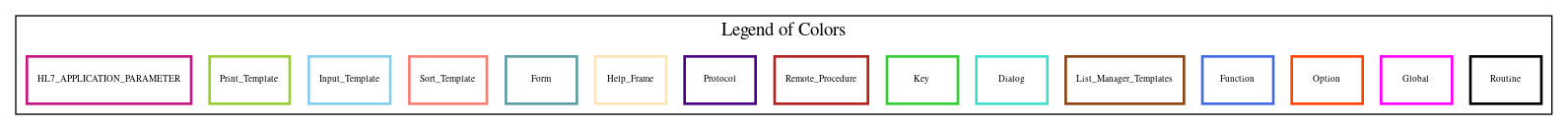Home   Package List   Routine Alphabetical List   Global Alphabetical List   FileMan Files List   FileMan Sub-Files List   Package Component Lists   Package-Namespace Mapping
Routine: LRBLAUD1

# Routine: LRBLAUD1

## Information

LRBLAUD1 ;TOG/CYM -AUDIT TRAIL UTILITY ;4/30/97 14:00

## Source Information

Source file <LRBLAUD1.m>

## Call Graph### Call Graph Total: 1

Package Total Call Graph
Lab Service 1 EN^LRUD

## Caller Graph

### Legends:#### Package Component Superscript legend

 action A extended action Ea event driver Ed subscriber Su protocol O limited protocol LP run routine RR broker B edit E server Se print P screenman SM inquire I### Caller Graph Total: 3

Package Total Caller Graph
Lab Service 3 LRBLIDTM  LRBLJED  LRBLJM1

## Entry Points

DISP ; When editing Unit Disposition, gets
; all associated data in files 65 and 63 that are also cleaned up
; and saves variables in the case the Disposition is Edited and the
; appropriate patient's transfusion record needs to be cleaned up.
; If so, these entries are then added to the audit trail.
DISP1 ; Actual code that adds data removed from the system when a
; disposition is deleted when using the option LRBLSED.
AUDIT
K ; Kills variables created during editing of a disposition
DISP4 ; Actual code used to evaluate when the DISPOSITION field (4.1)
; is edited and the software edits/deletes associated
; fields. Each field is evaluated and if there is a change
; the changes are captured on the audit trail.
DISP5 ; If Disposition is edited to TRANSFUSE, routine LRBLJED creates
; a patient transfusion record in file 63. Following code adds
; those changes to the audit trail.
MOD ; At the beginning of an edit session, collects all data
; in the MODIFIED TO/FROM field multiple, puts into a
; BEGM() array and counts total for later comparison.
MOD2 ; If a disposition of MODIFY is deleted, collects all data in the
; MODIFY TO/FROM field multiple (from the BEGM() array), and adds
; to the audit trail before the software deletes the entries.
MOD3 ; Counts MODIFY TO/FROM entries after unit is modified.
; If total entries after modification < total entries before
; modification puts deleted entry onto the audit trail
CHECK

## External References

Name Field # of Occurrence
EN^LRUD DISP1+4, AUDITMOD3+18, MOD3+19, CHECK

## Global Variables Directly Accessed

Name Line Occurrences  (* Changed,  ! Killed)
^LR - [#63] DISP+8, DISP5+3
^LRD(65 - [#65] DISP+5, DISP+6, DISP1+2, DISP4+4, DISP4+8, MOD+4, MOD2+4, MOD2+5, MOD3+4, MOD3+8
MOD3+17

## Label References

Name Line Occurrences
AUDIT DISP1+3, DISP1+5, DISP1+6, DISP1+7, DISP1+8, DISP1+9, DISP1+10, DISP1+11, DISP1+12, DISP1+13
DISP1+15, DISP1+16, DISP1+17, DISP1+18, DISP1+19, DISP1+20, DISP1+21, DISP1+22, DISP1+23, DISP1+24
DISP1+25, MOD2+6, MOD2+7, MOD2+8, MOD3+10, MOD3+11, MOD3+12
CHECK DISP4+5, DISP4+6, DISP4+7, DISP4+9, DISP4+10, DISP4+11, DISP4+12, DISP4+13, DISP4+14, DISP4+15
DISP4+16, DISP5+4, DISP5+5, DISP5+6, DISP5+7, DISP5+8, DISP5+9, DISP5+10, DISP5+11, DISP5+12
DISP5+13, DISP5+14
DISP5 DISP4+17

## Local Variables

### Legend:

 >> Not killed explicitly * Changed ! Killed ~ Newed

Name Field # of Occurrence
>> AFTM( MOD3+4*, MOD3+15
AFTMOD K+1!, MOD3+3*, MOD3+4*, MOD3+5, MOD3+13
>> AUD MOD3+6*, MOD3+7*, MOD3+8, MOD3+9, MOD3+14*, MOD3+15*, MOD3+16, MOD3+17
BEGM K+1!, MOD2+3
BEGM( MOD+4*, MOD3+7, MOD3+9, MOD3+16
BEGMOD K+1!, MOD+3*, MOD+4*, MOD3+5, MOD3+13
>> DA DISP+5, DISP+6, DISP1+2, DISP1+15*, DISP4+4, DISP4+8, DISP5+4*, MOD2+4
>> DA(1 DISP1+15*, DISP5+4*, MOD2+4*
DUZ DISP4+6
LRCOMP DISP+8*, DISP1+16, K+1!, DISP5+5
LRCOMPID DISP+8*, DISP1+17, K+1!, DISP5+6
LRDIPD DISP+5*, DISP1+5, K+1!, DISP4+7
LRDISDT DISP+5*, DISP1+3, K+1!, DISP4+5
LRDISP DISP+5*, K+1!
LRDIST K+1!
LRDSP DISP+5*, K+1!
LRENTP DISP+8*, DISP1+18, K+1!, DISP5+7
LRIEN K+1!, MOD+4, MOD2+4, MOD2+5, MOD3+4, MOD3+7, MOD3+8, MOD3+9, MOD3+15, MOD3+16
MOD3+17
>> LRM DISP4+4*, DISP4+5, DISP4+7, DISP4+8*, DISP4+9, DISP4+10, DISP4+11, DISP4+12, DISP4+13, DISP4+14
DISP4+15, DISP4+16, DISP5+3*, DISP5+4, DISP5+5, DISP5+6, DISP5+7, DISP5+8, DISP5+9, DISP5+10
DISP5+11, DISP5+12, DISP5+13, DISP5+14, MOD2+5*, MOD2+6, MOD2+7, MOD2+8, MOD3+9*, MOD3+10
MOD3+11, MOD3+12, MOD3+17*, MOD3+18, MOD3+19
LROLD DISP+9*, DISP1+23, K+1!, DISP5+12
LRPERS DISP+5*, DISP1+4, K+1!, DISP4+6
LRPHYS DISP+6*, DISP1+7, K+1!, DISP4+10
LRPOOL DISP+9*, DISP1+21, K+1!, DISP5+10
LRPROVN DISP+7*, DISP1+11, K+1!, DISP4+14
LRPTR DISP+6*, DISP+7, DISP+8, DISP1+6, DISP1+14, DISP1+15, K+1!, DISP4+9, DISP4+17, DISP5+3
DISP5+4
LRPTRANS DISP+6*, DISP+7, K+1!
LRPTREC DISP+8*, DISP+9, K+1!
LRREACT DISP+6*, DISP1+10, K+1!, DISP4+13
LRREC DISP+6*, DISP+8, DISP1+9, DISP1+15, K+1!, DISP4+12, DISP5+3, DISP5+4
LRRECRX DISP+9*, DISP1+22, K+1!, DISP5+11
LRRXTYPE DISP+7*, DISP1+13, K+1!, DISP4+16
LRTRDT DISP+8*, DISP1+15, K+1!, DISP5+4
LRTS DISP+6*, DISP1+8, K+1!, DISP4+11
LRTSNUM DISP+7*, DISP1+12, K+1!, DISP4+15
LRTYPE DISP+9*, DISP1+25, K+1!, DISP5+14
LRUNABO DISP+8*, DISP1+19, K+1!, DISP5+8
LRUNRH DISP+9*, DISP1+20, K+1!, DISP5+9
LRVOL DISP+9*, DISP1+24, K+1!, DISP5+13
MOD K+1!, MOD+3*, MOD+4*, MOD2+4*, MOD2+5, MOD3+3*, MOD3+4*
NODE2 K+1!
>> O DISP1+3*, DISP1+4*, DISP1+5*, DISP1+6*, DISP1+7*, DISP1+8*, DISP1+9*, DISP1+10*, DISP1+11*, DISP1+12*
DISP1+13*, DISP1+15*, DISP1+16*, DISP1+17*, DISP1+18*, DISP1+19*, DISP1+20*, DISP1+21*, DISP1+22*, DISP1+23*
DISP1+24*, DISP1+25*, AUDITDISP4+5*, DISP4+6*, DISP4+7*, DISP4+9*, DISP4+10*, DISP4+11*, DISP4+12*
DISP4+13*, DISP4+14*, DISP4+15*, DISP4+16*, DISP5+4*, DISP5+5*, DISP5+6*, DISP5+7*, DISP5+8*, DISP5+9*
DISP5+10*, DISP5+11*, DISP5+12*, DISP5+13*, DISP5+14*, MOD2+6*, MOD2+7*, MOD2+8*, MOD3+10*, MOD3+11*
MOD3+12*, MOD3+18*, MOD3+19*, CHECK
U DISP+5, DISP+6, DISP+7, DISP+8, DISP+9, DISP4+5, DISP4+7, DISP4+9, DISP4+10, DISP4+11
DISP4+12, DISP4+13, DISP4+14, DISP4+15, DISP4+16, DISP5+4, DISP5+5, DISP5+6, DISP5+7, DISP5+8
DISP5+9, DISP5+10, DISP5+11, DISP5+12, DISP5+13, DISP5+14, MOD2+6, MOD2+7, MOD2+8, MOD3+10
MOD3+11, MOD3+12, MOD3+18, MOD3+19
>> X DISP1+4*, AUDIT*, DISP4+5*, DISP4+6*, DISP4+7*, DISP4+9*, DISP4+10*, DISP4+11*, DISP4+12*, DISP4+13*
DISP4+14*, DISP4+15*, DISP4+16*, DISP5+4*, DISP5+5*, DISP5+6*, DISP5+7*, DISP5+8*, DISP5+9*, DISP5+10*
DISP5+11*, DISP5+12*, DISP5+13*, DISP5+14*, MOD3+18*, MOD3+19*, CHECK
>> Z DISP1+3*, DISP1+4*, DISP1+5*, DISP1+6*, DISP1+7*, DISP1+8*, DISP1+9*, DISP1+10*, DISP1+11*, DISP1+12*
DISP1+13*, DISP1+15*, DISP1+16*, DISP1+17*, DISP1+18*, DISP1+19*, DISP1+20*, DISP1+21*, DISP1+22*, DISP1+23*
DISP1+24*, DISP1+25*, DISP4+5*, DISP4+6*, DISP4+7*, DISP4+9*, DISP4+10*, DISP4+11*, DISP4+12*, DISP4+13*
DISP4+14*, DISP4+15*, DISP4+16*, DISP5+4*, DISP5+5*, DISP5+6*, DISP5+7*, DISP5+8*, DISP5+9*, DISP5+10*
DISP5+11*, DISP5+12*, DISP5+13*, DISP5+14*, MOD2+6*, MOD2+7*, MOD2+8*, MOD3+10*, MOD3+11*, MOD3+12*
MOD3+18*, MOD3+19*